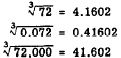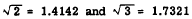Custom SearchTABLES OF ROOTS The decimal values of square roots and cube roots of numbers with as many as 3 or 4 digits can be found from tables. The table in appendix I of this course gives the square roots and cube roots of numbers from 1 to 100. Most of the values given in such tables are approximate numbers which have been rounded off. For example the fourth column in appendix I shows that= 8.4853, to 4 decimal places. By shifting the decimal point we can obtain other square roots. A shift of two places in the decimal point in the radicand corresponds to a shift of one place in the same direction in the square root. The following examples show the effect, as reflected in the square root, of shifting the location of the decimal point in the number whose square root we seek:Cube Root The fifth column in appendix I shows that the cube root of 72 is 4.1602. By shifting the decimal point we immediately have the cube roots of certain other numbers involving the same digits. A shift of three places in the decimal point in the radicand corresponds to a shift of one place in the same direction in the cube root. Compare the following examples:Many irrational numbers in their simplified forms involveandSince these radicals occur often, it is convenient to remember their decimal equivalents as follows:Thus any irrational numbers that do not contain any radicals other thanorcan be converted to decimal forms quickly without referring to tables. For example considerKeep in mind that the decimal equivalents ofandas used in the foregoing examples are not exact numbers and the results obtained with them are approximate in the fourth decimal place.